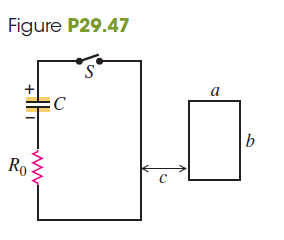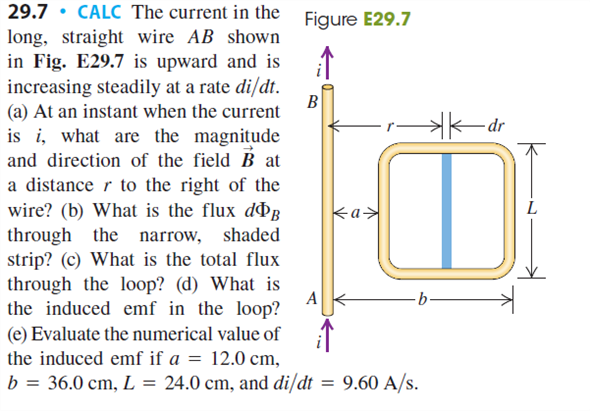Problem

# CP CALC In the circuit shown in Fig. P29.47, the capacitor has capacitance C = 20 µF...

CP CALC In the circuit shown in Fig. P29.47, the capacitor has capacitance C = 20 µF and is initially charged to 100 V with the polarity shown. The resistor R0 has resistance 10 Ω. At time t = 0 the switch S is closed. The small circuit is not connected in any way to the large one. The wire of the small circuit has a resistance of 1.0 Ω/m and contains 25 loops. The large circuit is a rectangle 2.0 m by 4.0 m, while the small one has dimensions a = 10.0 cm and b = 20.0 cm. The distance c is 5.0 cm. (The figure is not drawn to scale.) Both circuits are held stationary. Assume that only the wire nearest the small circuit produces an appreciable magnetic field through it. (a) Find the current in the large circuit 200 µs after S is closed. (b) Find the current in the small circuit 200 µs after S is closed. (Hint: See Exercise 29.7.) (c) Find the direction of the current in the small circuit. (d) Justify why we can ignore the magnetic field from all the wires of the large circuit except for the wire closest to the small circuit.#### Step-by-Step Solution

Request Professional Solution

#### Request Solution!

We need at least 10 more requests to produce the solution.

0 / 10 have requested this problem solution

The more requests, the faster the answer.

All students who have requested the solution will be notified once they are available.